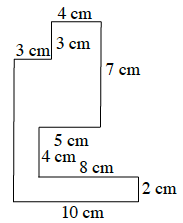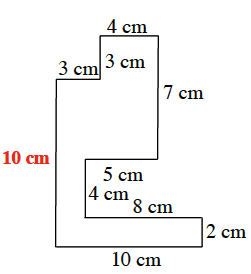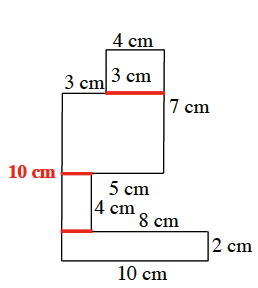Home > CC1 > Chapter 8 > Lesson 8.2.1 > Problem8-81

8-81.

Find the area and perimeter of the figure shown below. All angles are right angles. Show your work clearly. Homework Help ✎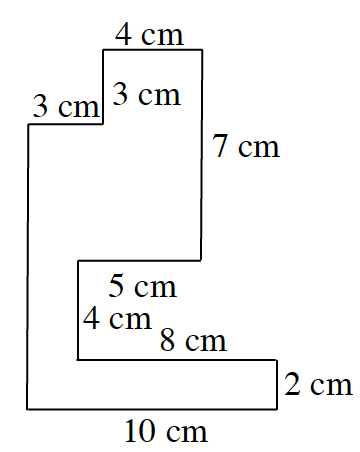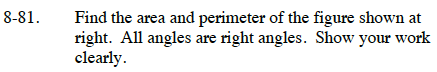Find the missing side.

Add up all the sides to find the perimeter.

The perimeter is 56 cm.

Break up the figure into rectangles.

Find the area of each rectangle and add them together to find the area of the figure.

20 sq cm + 8 sq cm + 28 sq cm + 12 sq cm = 68 sq cm